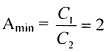Courses

# Analog Electronics - MCQ Test - 1

## 15 Questions MCQ Test GATE ECE (Electronics) 2022 Mock Test Series | Analog Electronics - MCQ Test - 1

Description
This mock test of Analog Electronics - MCQ Test - 1 for Electronics and Communication Engineering (ECE) helps you for every Electronics and Communication Engineering (ECE) entrance exam. This contains 15 Multiple Choice Questions for Electronics and Communication Engineering (ECE) Analog Electronics - MCQ Test - 1 (mcq) to study with solutions a complete question bank. The solved questions answers in this Analog Electronics - MCQ Test - 1 quiz give you a good mix of easy questions and tough questions. Electronics and Communication Engineering (ECE) students definitely take this Analog Electronics - MCQ Test - 1 exercise for a better result in the exam. You can find other Analog Electronics - MCQ Test - 1 extra questions, long questions & short questions for Electronics and Communication Engineering (ECE) on EduRev as well by searching above.
QUESTION: 1

### If OPAMP’s used in circuit shown are ideal then given circuit acts as: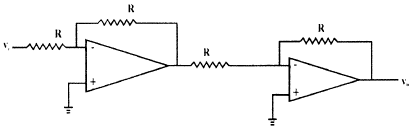Solution:

Here vo = Vi so it is working like a voltage follower. Voltage follower is a non-inverting amplifier and is used as buffer.

QUESTION: 2

### For audio frequency applications, the popular oscillator used is

Solution:

Characteristics like ease of tuning, very low distortion and good frequency stability of Wien bridge oscillator makes it becomes popular for audio frequency applications. Phase shift oscillator and Wien bridge oscillator both can be used as audio frequency range. But Wien bridge oscillator can be used for very wide frequency range.

QUESTION: 3

### A differential amplifier has inputs v1 = 1050 µV and v2 = 950 µV, with CMRR = 100, what is the error in differential output:

Solution:
QUESTION: 4

A class B Push pull amplifier with transformer coupled load uses two transistors rated 10W each what is maximum power output one can obtain at the load from circuit.

Solution:
QUESTION: 5

If the input to the ideal comparator shown in the figure is a sinusoidal signal of 8 V (peak to peak) without any DC component, then the output of the comparator has a duty cycle of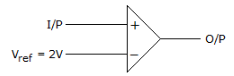Solution:
QUESTION: 6

A 12 V monolithic regulator is adjusted to obtain a higher output voltage as shown in fig, then V0 is: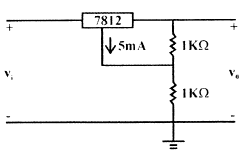Solution: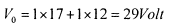*Answer can only contain numeric values
QUESTION: 7

For a transistor amplifier with self biasing network the following components are used.

R1 = 4K, R2 = 4K, Re = 1 KΩ, then the value of stability factor (S) is ________.

Solution: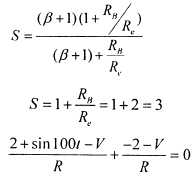QUESTION: 8

For the circuit shown in figure assume β = 100, for the transistor, the Si transistor will be in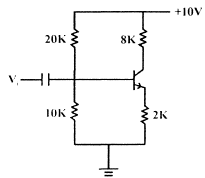Solution:
QUESTION: 9

What is hysteresis voltage for a given Schmitt trigger circuit?
→ Vsat = ± 10 VoltSolution:QUESTION: 10

Feedback factor (β) of circuit is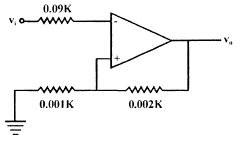Solution: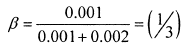QUESTION: 11

Voltage Series feedback also called series-shunt feedback) results in

Solution:

QUESTION: 12

If given diode is ideal, then value of I is.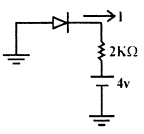Solution:

Diode is R.biased

QUESTION: 13

In an amplifier with feedback following effects were observed Voltage gain (↓), input impedance (↑), output impedance (↓) and distortion (↓), this is a case of

Solution:
QUESTION: 14

A sinusoidal input voltage of frequency ω is fed to circuit shown in figure if C1 >> C2 and Vm is the peak value of input voltage, then what is the value of output voltage?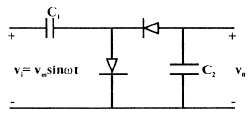Solution:

It is a Clamper circuit which is nothing but voltage doubler circuit.

QUESTION: 15

For given colpitt oscillator, if C1 = 600 pF, C2 = 300 pF and L = 50 µH, then the minimum value of gain to maintain oscillation is: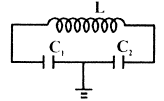Solution: